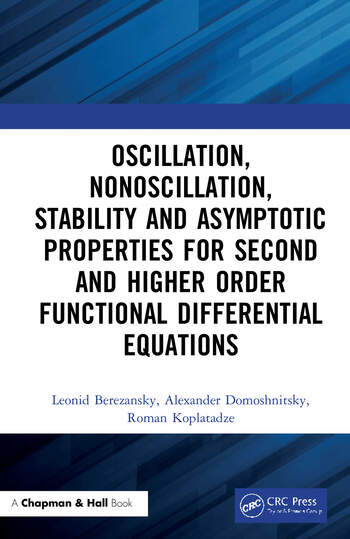# Oscillation, Nonoscillation, Stability and Asymptotic Properties for Second and Higher Order Functional Differential Equations

## 1st Edition

Alexander Domoshnitsky, Leonid Berezansky, Roman Koplatadz

Chapman and Hall/CRC
May 8, 2020 Forthcoming
Reference - 590 Pages
ISBN 9780367337544 - CAT# 317236

USD\$220.00

Available for pre-order. Item will ship after May 8, 2020
FREE Standard Shipping!

## Preview

### Summary

Asymptotic properties of solutions such as stability/ instability,oscillation/ nonoscillation, existence of solutions with specific asymptotic, maximum principles present a classical part in the theory of higher order functional differential equations. The use of these equations in applications is one of the main reasons for the developments in this field. The control in the mechanical processes leads to mathematical models with second order delay differential equations. Stability and stabilization of second order delay equations are one of the main goals of this book. The book is based on the authors’ results in the last decade.

Features:

• Stability, oscillatory and asymptotic properties of solutions are studied in correlation with each other.
• The first systematic description of stability methods based on Bohl-Perron theorem.
• Simple and explicit exponential stability tests.

In this book, various types of functional differential equations are considered: second and higher orders delay differential equations with measurable coefficients and delays, integro-differential equations, neutral equations, operator equations. Oscillation/nonoscillation, existence of unbounded solutions, instability, special asymptotic behavior, positivity, exponential stability and stabilization of functional differential equations are studied. New methods for the study of exponential stability are proposed. Note among them such as the W-transform (right regularization), a priory estimation of solutions, maximum principles, differential and integral inequalities, matrix inequality method, reduction to a system of equations.

The book can be used by applied mathematicians and as a basis for a course on stability of functional differential equations for graduate students.